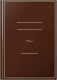# Transactions of the Cambridge Philosophical SocietyCambridge University Press, 1804

### „« ÌÞÊ·Â «·‰«” -þ «»… „—«Ã⁄…

·„ ‰⁄À— ⁄·Ï √Ì „—«Ã⁄«  ðÌ «·√„«þ‰ «·„⁄ «œ….

### «·„Õ ÊÌ«

 On Differential Equations with two Independent Variables By A C Dixon Sc D 1 On the Calculation of the DoubleIntegral expressing Normal Correlation By W 23 On the simplest Algebraic Minimal Curves and the derived Real Minimal Surfaces 69 Diophantine Inequalities By G B Mathews M A F R S 83 The Diophantine Inequality kc fxy By Major P A MacMahon R A D 111 Rational SpaceCurves of the Fourth Order By Herbert Richmond M A Kings 132 On Divergent Hypergeometric Series By W MF Orr Professor of Mathematics 151 On the Fifth Book of Euclids Elements Second Paper By M J M Hill M A 157
 The case of three intersecting double lines two of which coincide 263 The case of a curve of multiplicity greater than 2 264 811 269 QuiNTICS WITH TWO LINEARLY INDEPENDENT INTEGRALS OP THE FIRST KIND WHICH ARE FUNCTIONS OF ONE ANOTHER 8 Preli... 271 Integration of the differential equation 274 Summary of Part II 282 The CASE OF two functionally independent integrals of the first kind 12 Preliminary 283 Discussion of possible singularities 284

 Some Problems in Electric Convection By Gilbert T Walker M A Fellow 173 On a class of Matrices of infinite order ami on the existence of matricial functions 190 Seminvariants of Systems of Binary Qualities the order of each quautic being infinite 234 Introduction 250 27 251 Preliminary classification of quintic surfaces 252 The case of a double quintic curve 253 The case of a double quadriquadric 254 The case of three or more intersecting double straight lines 257
 The case of an ordinary conical double point 285 15 The case of a biplanar double point 286 16 The case of a uniplanar double point 287 The case of a double straight line 291 On Differentiation and Integration of Divergent Series By G H Hardy M A 297 On the Classificatioii of Integral Functions By E W Barnes M A Fellow 322 On the rise of a Spinning Top By E G Gallop M A Fellow of Gonville 356 On the Theory of the Multiple Gamma Function By E W Barnes M A 374 On the Asymptotic Expansion of Integral Functions of Multiple Linear Sequence 426